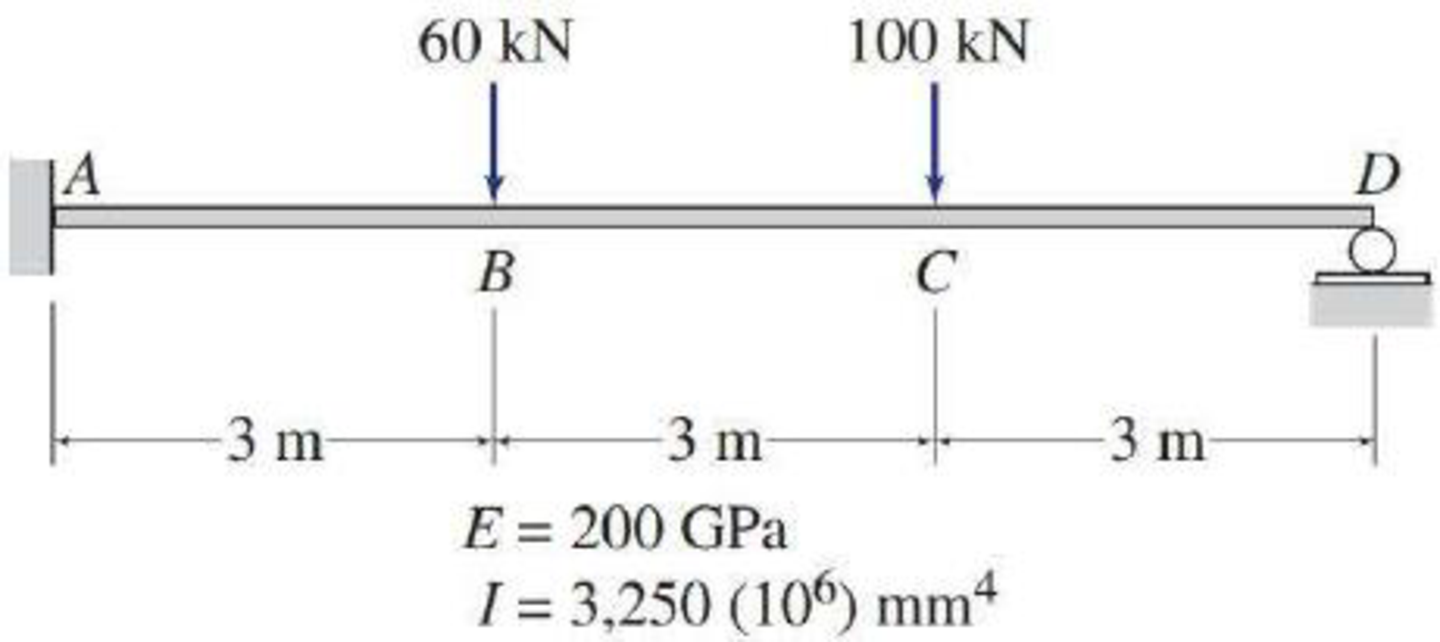# Solve Problem 13.1 for the loading shown and a settlement of 30 mm at support D . See Fig. P13.1. 13.1 through 13.4 Determine the reactions and draw the shear and bending moment diagrams for the beams shown in Figs. P13.1–P13.4 using the method of consistent deformations. Select the reaction at the roller support to be the redundant. FIG. P13.1, P13.5, P13.49

#### Solutions

Chapter
Section
Chapter 13, Problem 49P
Textbook Problem
38 views

## Solve Problem 13.1 for the loading shown and a settlement of 30 mm at support D. See Fig. P13.1.13.1 through 13.4 Determine the reactions and draw the shear and bending moment diagrams for the beams shown in Figs. P13.1–P13.4 using the method of consistent deformations. Select the reaction at the roller support to be the redundant.FIG. P13.1, P13.5, P13.49To determine

Calculate the reactions and sketch the shear and bending moment diagrams for the given beam.

### Explanation of Solution

Given information:

The settlement at support D (ΔD) is 30 mm.

Apply the sign conventions for calculating reactions, forces, and moments using the three equations of equilibrium as shown below.

• For summation of forces along x-direction is equal to zero (Fx=0), consider the forces acting towards right side as positive (+) and the forces acting towards left side as negative ().
• For summation of forces along y-direction is equal to zero (Fy=0), consider the upward force as positive (+) and the downward force as negative ().
• For summation of moment about a point is equal to zero (Matapoint=0), consider the clockwise moment as negative and the counter clockwise moment as positive.

Calculation:

Show the free body diagram of the given beam as shown in Figure 1.

Refer Figure 1.

Consider the horizontal and vertical reaction at A are denoted by Ax and Ay.

Consider the moment at A is denoted by MA.

Consider the vertical reaction at D is denoted by Dy.

The reactions acting in the beam is 4.

The number of Equilibrium reaction is 3. Then,

The degree of indeterminacy of the beam is 1.

Take the vertical reaction at D as the redundant.

Modify the Figure 1 as shown in Figure 2.

Refer Figure 2.

The deflection at D due to the external moment is denoted by ΔD.

The deflection coefficient representing the deflection at D due to unit value of redundant Dy is fDD.

The deflection at D due to unknown redundant Dy is fDD.

Show the deflection at the free end of the cantilever beam with a point load P acting at a distance from the fixed end as shown below.

Δ=Pa26EI(3la)        (1)

Here, l is the length of the beam, E is modulus of Elasticity of the beam, I is the moment of inertia.

Calculate the deflection at the free end of the beam due to the load of 60kN as shown below.

Substitute 3m for a, 9m for l, and 60kN for P in Equation (1).

Δ1=60×326EI(3×93)=2160EI

Calculate the deflection at the free end of the beam due to the load of 100kN as shown below.

Substitute 6m for a, 9m for l, and 100kN for P in Equation (1).

Δ2=100×626EI(3×96)=12600EI

Calculate the deflection at D due to the loading on the beam as shown below.

ΔD0=Δ1+Δ2=2,160EI12,600EI=14,760kNm3EI        (2)

Substitute 200GPa for E and 3,250×106mm4 for I in Equation (2).

ΔD0=14,760kNm3200GPa×106kN/m21GPa×3,250×106mm4×(1m1,000mm)4=14,760650,000=0.0227m

Calculate the value of fDD as shown below.

Substitute 9m for a, 9m for l, and 1kN for P in Equation (1).

fDD=1×926EI(3×99)=243kNm3/kNEI        (3)

Substitute 200GPa for E and 3,250×106mm4 for I in Equation (3).

fDD=243kNm3/kN200GPa×106kN/m21GPa×3,250×106mm4×(1m1,000mm)4=243650,000=0

### Still sussing out bartleby?

Check out a sample textbook solution.

See a sample solution

#### The Solution to Your Study Problems

Bartleby provides explanations to thousands of textbook problems written by our experts, many with advanced degrees!

Get Started

Find more solutions based on key concepts
Convert the results of Example 11.13 from Btu to calories.

Engineering Fundamentals: An Introduction to Engineering (MindTap Course List)

How can mobile security apps protect your mobile device data? (237)

Enhanced Discovering Computers 2017 (Shelly Cashman Series) (MindTap Course List)

If your motherboard supports ECC DDR3 memory, can you substitute non-ECC DDR3 memory?

A+ Guide to Hardware (Standalone Book) (MindTap Course List)